# Pointer Basics in C

Last updated on July 27, 2020

The real power of C lies in pointers. The pointers are slightly difficult to grasp at first. After going through the basics of pointers, you will get a better idea about what they are and how to use them.

## What is a Pointer? #

A pointer is a variable used to store a memory address. Let's first learn how memory is organized inside a computer.

Memory in a computer is made up of bytes (A byte consists of 8 bits) arranged in a sequential manner. Each byte has a number associated with it just like index or subscript in an array, which is called the address of the byte. The address of byte starts from 0 to one less than the size of memory. For example, say in a 64MB of RAM, there are 64 * 2^20 = 67108864 bytes. Therefore the address of these bytes will start from 0 to 67108863.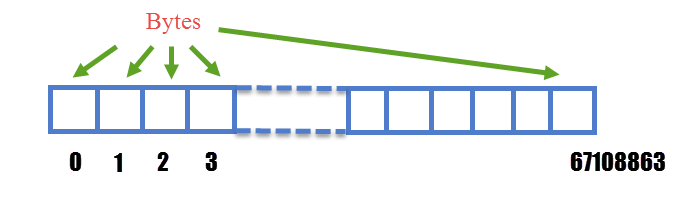Let's see what happens when you declare a variable.

int marks;


As we know an int occupies 4 bytes of data (assuming we are using a 32-bit compiler) , so compiler reserves 4 consecutive bytes from memory to store an integer value. The address of the first byte of the 4 allocated bytes is known as the address of the variable marks. Let's say that address of 4 consecutive bytes are 5004, 5005, 5006 and 5007 then the address of the variable marks will be 5004.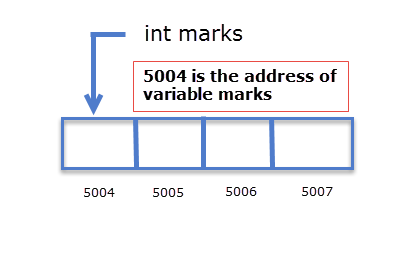To find the address of a variable, C provides an operator called address operator (&). To find out the address of the variable marks we need to place & operator in front of it, like this:

&marks


The following program demonstrates how to use the address operator (&).

  1 2 3 4 5 6 7 8 9 10 11 12 13 14 // Program to demonstrate address(&) operator #include int main() { int i = 12; printf("Address of i = %u \n", &i); printf("Value of i = %d ", i); // signal to operating system program ran fine return 0; } 

Expected Output:

 1 2 Address of i = 2293340 Value of i = 12 

Note: Address of i may vary every time you run the program.

How it works:

To find the address of the variable, precede the variable name by & operator. Another important thing to notice about the program is the use of %u conversion specification. Recall that %u conversion specification is used to print unsigned decimal numbers and since the memory addresses can't be negative, you must always use %u instead of %d.

Address of operator (&) can't be used with constants or expression, it can only be used with a variable.

 1 2 3 4 5 &var; // ok &12; // error because we are using & operator with a constant &(x+y) // error because we are using & operator with an expression 

We have been using address operator(&) in the function scanf() without knowing why? The address of a variable is provided to scanf(), so that it knows where to write data.

## Declaring Pointer Variables #

As already said a pointer is a variable that stores a memory address. Just like any other variables you need to first declare a pointer variable before you can use it. Here is how you can declare a pointer variable.

Syntax: data_type *pointer_name;

data_type is the type of the pointer (also known as the base type of the pointer).
pointer_name is the name of the variable, which can be any valid C identifier. Let's take some examples:

 1 2 int *ip; float *fp; 

int *ip means that ip is a pointer variable capable of pointing to variables of type int. In other words, a pointer variable ip can store the address of variables of type int only. Similarly, the pointer variable fp can only store the address of a variable of type float. The type of variable (also known as base type) ip is a pointer to int and type of fp is a pointer to float. A pointer variable of type pointer to int can be symbolically represented as (int *). Similarly, a pointer variable of type pointer to float can be represented as (float *).

Just like other variables, a pointer is a variable so, the compiler will reserve some space in memory. All pointer variable irrespective of their base type will occupy the same space in memory. Normally 4 bytes or 2 bytes (On a 16-bit Compiler) are used to store a pointer variable (this may vary from system to system).

## Assigning Address to Pointer Variable #

After declaring a pointer variable the next step is to assign some valid memory address to it. You should never use a pointer variable without assigning some valid memory address to it, because just after declaration it contains garbage value and it may be pointing to anywhere in the memory. The use of an unassigned pointer may give an unpredictable result. It may even cause the program to crash.

 1 2 3 4 5 int *ip, i = 10; float *fp, f = 12.2; ip = &i; fp = &f; 

Here ip is declared as a pointer to int, so it can only point to the memory address of an int variable. Similarly, fp can only point to the address of a float variable. In the last two statements, we have assigned the address of i and f to ip and fp respectively. Now, ip points to the variable i and fp points to variable f. It is important to note that even if you assign an address of a float variable to an int pointer, the compiler will not show you any error but you may not get the desired result. So as a general rule always you should always assign the address of a variable to its corresponding pointer variable of the same type.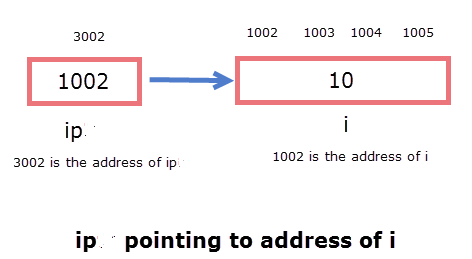We can initialize the pointer variable at the time of declaration, but in this case, the variable must be declared and initialized before the pointer variable.

int i = 10, *iptr = &i;


You can assign the value of one pointer variable to another pointer variable If their base type is same. For example:

 1 2 3 4 5 int marks = 100, *p1, *p2; p1 = &marks; p2 = p1; 

After the assignment, p1 and p2 points to the same variable marks.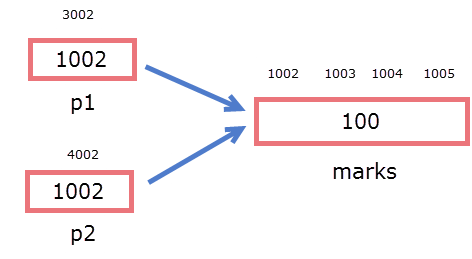As already said when a pointer variable is declared it contains garbage value and it may be point anywhere in the memory. You can assign a symbolic constant called NULL (defined in stdio.h) to any pointer variable. The assignment of NULL guarantees that the pointer doesn't point to any valid memory location.

 1 2 3 int i = 100, *iptr; iptr = NULL; 

## Dereferencing Pointer Variable #

Dereferencing a pointer variable simply means accessing data at the address stored in the pointer variable. Up until now, we have been using the name of the variable to access data inside it, but we can also access variable data indirectly using pointers. To make it happen, we will use a new operator called the indirection operator (*). By placing indirection operator (*) before a pointer variable we can access data of the variable whose address is stored in the pointer variable.

int i = 100, *ip = &i;


Here ip stores address of variable i, if we place * before ip then we can access data stored in the variable i. It means the following two statements does the same thing.

 1 2 printf("%d\n", *ip); // prints 100 printf("%d\n", i); // prints 100 

Indirection operator (*) can be read as value at the address. For example, *ip can be read as value at address ip.

Note: It is advised that you must never apply indirection operator to an uninitialized pointer variable, doing so may cause unexpected behaviour or the program may even crash.

 1 2 int *ip; printf("%d", *ip); // WRONG 

Now we know by dereferencing a pointer variable, we can access the value at the address stored in the pointer variable. Let's dig a little deeper to view how the compiler actually retrieves data.

 1 2 3 4 5 6 7 char ch = 'a'; int i = 10; double d = 100.21; char *cp = &ch; int *ip = &i; double *ip = &d; 

Let's say pointer cp contains the address 1000. When we write *cp the compiler knows that it has to retrieve information from the starting address 1000. Now the question arises how much data to retrieve from starting address 1000 ? 1 bytes, 2 bytes; What do you think ? To know how much information to retrieve from starting address 1000, the compiler looks at the base type of pointer and will retrieve the information depending upon the base type of pointer. For example, if the base type is pointer to char then 1 byte of information from the starting address will be retrieved and if the base type pointer to int then 4 bytes of information from the starting address will be retrieved. It is important to note that if you are on a system where the size of int is 2 bytes then 2 bytes of information from the starting address will be retrieved.

So in our case, only 1 byte of data from starting address will be retrieved. i.e the data stored at address 2000 will be retrieved only.

Similarly, if ip points to the address 2000. On writing *ip compiler will retrieve 4 bytes of data starting from address 2000.

In the following image, shaded portion shows the number of bytes retrieved.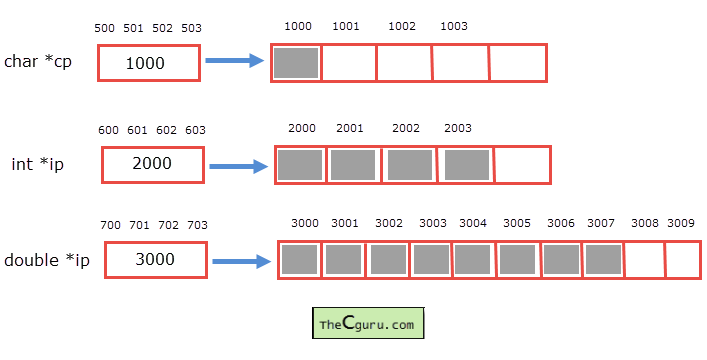Before moving ahead, Interpret the meaning of the following expression:

*(&i) , where i is a variable of type int.

We know from the precedence table that parentheses () has the highest precedence, so &i is evaluated first. Since &i is the address of variable i, so dereferencing it with * operator will give us the value of the variable i. So we can conclude that writing *(&i) is same as writing i.

The following example demonstrates everything we have learned about pointers so far.

  1 2 3 4 5 6 7 8 9 10 11 12 13 14 15 16 17 18 19 20 21 22 23 24 25 #include int main() { int i = 12, *ip = &i; double d = 2.31, *dp = &d; printf("Value of ip = address of i = %d\n", ip); printf("Value of fp = address of d = %d\n\n", d); printf("Address of ip = %d\n", &ip); printf("Address of dp = %d\n\n", &dp); printf("Value at address stored in ip = value of i = %d\n", *ip); printf("Value at address stored in dp = value of d = %f\n\n", *dp); // memory occupied by pointer variables // is same regardless of its base type printf("Size of pointer ip = %d\n", sizeof(ip)); printf("Size of pointer dp = %d\n\n", sizeof(dp)); // signal to operating system program ran fine return 0; } 

Expected Output:

  1 2 3 4 5 6 7 8 9 10 11 12 Value of ip = address of i = 2686788 Value of fp = address of d = 1202590843 Address of ip = 2686784 Address of dp = 2686772 Value at address stored in ip = value of i = 12 Value at address stored in dp = value of d = 2.310000 Size of pointer ip = 4 Size of pointer dp = 4 

Note: Memory address may vary every time you run the program.

There is nothing new in the above program that deserves any explanation. Before we proceed to next chapter, always remember that the size of the pointer variables is same regardless of its base type but the size of the memory address that will be accessed while dereferencing depends on upon the base type of the pointer variable.